# Basic validation: numeric

Multiple choice

Which one of these would most accurately detect a spider?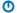A
• If tSpinsWeb = "yes" Or sNumLegs < 10 Then
•     MsgBox "It's a spider."
• EndIf
B
• If Not (tSpinsWeb = "yes" And sNumLegs <> 8) Then
•     MsgBox "It's a spider."
• EndIf
C
• If tSpinsWeb = "yes" And Not (sNumLegs <> 8) Then
•     MsgBox "It's a spider."
• EndIf
D
• If Not (tSpinsWeb = "yes" And sNumLegs = 8) Then
•     MsgBox "It's a spider."
• EndIf

Not graded. So why do it?

Lesson contents

Validating numbers is much like strings, but with an extra twist.

### Type mismatch

Let's go back to computing a restaurant tip. Let's say we made a worksheet like this: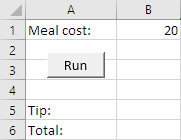Here's the code:

• Sub runClicked()
•
•   Dim sMealCost As Single
•   Dim sTipAmount As Single
•   Dim sTotal As Single
•
•   sMealCost = Cells(1, 2)
•   sTipAmount = sMealCost * 0.15
•   sTotal = sMealCost + sTipAmount
•   Cells(5, 2) = sTipAmount
•   Cells(6, 2) = sTotal
•
• End SubRay

This code looks easier than it seemed a couple of weeks ago.

Good point. You've learned. You're closer to the Benjamins.

OK, let's put a word for the mean cost.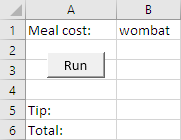What's going to happen when the program runs?

Reflect

Run your own tip program. What happens when there's a word for meal cost?

If you were logged in as a student, the lesson would pause here, and you'd be asked to type in a response. If you want to try that out, ask for an account on this site.Georgina

The program crashes. Here's the error message: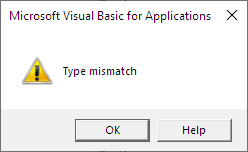A type mismatch has to do with a variable's data type. Here's the code:

• Dim sMealCost As Single
•   ...
•   sMealCost = Cells(1, 2)

`sMealCost` is a `Single`. That's it's data type. It can hold only numbers. `Cells(1, 2)` has text in it. Try to put text in a numeric variable, and you get a type mismatch crash.

### Preventing type mismatches

Let's rewrite the code to get around the problem. Trying to put text into a `Single` variable causes the crash. But we can put anything we like into a `String` variable. Then, we can check the contents of the `String` variable, to see if it's a number.

• 'A temporary variable to hold unchecked user input.
• Dim tUncheckedUserInput as String
• Dim sMealCost As Single
• ...
• tUncheckedUserInput = Cells(1, 2)

It won't crash, because `tUncheckedUserInput` is a string, and any data can go into a string.

Now let's check `tUncheckedUserInput`.

• 'A temporary variable to hold unchecked user input.
• Dim tUncheckedUserInput as String
• Dim sMealCost As Single
• ...
• tUncheckedUserInput = Cells(1, 2)
• If Not IsNumeric(tUncheckedUserInput) Then
•     MsgBox "Sorry, meal cost must be a number."
•     End
• End If

The `IsNumeric()` function returns true or false. True if what you give it is a valid number, false if it isn't. So, if the user types a word in `Cells(1, 2)`, the user gets an error message, and the program stops.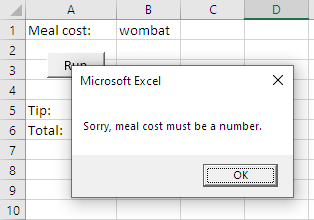This is a friendly error message, that tells the user what they need to fix.

One more thing to do. Move the user's input, that we've now checked, into a numeric variable, so we can do calculations with it.

• 'A temporary variable to hold unchecked user input.
• Dim tUncheckedUserInput as String
• Dim sMealCost As Single
• ...
• tUncheckedUserInput = Cells(1, 2)
• If Not IsNumeric(tUncheckedUserInput) Then
•     MsgBox "Sorry, meal cost must be a number."
•     End
• End If
• sMealCost = tUncheckedUserInput

This is safe, because we know that `tUncheckedUserInput` has a number in it. So copying the number to `sMealCost` won't cause a crash.

We can put that into a pattern.

Pattern

Put a value into a string. Use the `IsNumeric()` function.

### Range check

Usually, you need more than one validation check on numeric data. There are at least two validation rules for meal cost:

• Meal cost must be a number.
• Meal cost cannot be negative.

We need to have both checks, to validate what the user enters for meal cost.

We need a range check, that is, making sure that the number isn't too small, or too large.

• 'A temporary variable to hold unchecked user input.
• Dim tUncheckedUserInput as String
• Dim sMealCost As Single
• ...
• tUncheckedUserInput = Cells(1, 2)
• If Not IsNumeric(tUncheckedUserInput) Then
•     MsgBox "Sorry, meal cost must be a number."
•     End
• End If
• ' tUncheckedUserInput is numeric, so move its value into a Single.
• sMealCost = tUncheckedUserInput
• ' Range check.
• If sMealCost < 0 Then
•     MsgBox "Sorry, meal cost cannot be negative."
•     End
• End If
Pattern

Check whether a number is between two other numbers.

Warning: "range" has two meanings. This pattern is not about `Range` objects.

### The full pattern

Now we have our full numeric validation pattern. It uses the type check pattern, and usually, but not always, the range check pattern.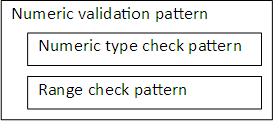The numeric validation pattern is two other patterns stuck together.

We'll be improving this pattern soon.

### Reusing the temporary variable

Once we've done this...

• sMealCost = tUncheckedUserInput

... we don't need the data in `tUncheckedUserInput` any more. We can reuse the variable, if we want.

Let's say the user gets to type in the tip rate, like this: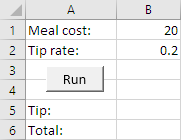We need to validate two numeric values now. Here's some code.

• 'Validate meal cost
• tUncheckedUserInput = Cells(1, 2)
• If Not IsNumeric(tUncheckedUserInput) Then
•     MsgBox "Sorry, meal cost must be a number."
•     End
• End If
• sMealCost = tUncheckedUserInput
• If sMealCost < 0 Then
•     MsgBox "Sorry, meal cost cannot be negative."
•     End
• End If
•
• 'Validate tip rate
• tUncheckedUserInput = Cells(2, 2)
• If Not IsNumeric(tUncheckedUserInput) Then
•     MsgBox "Sorry, tip rate must be a number."
•     End
• End If
• sTipRate = tUncheckedUserInput
• If sTipRate < 0 Then
•     MsgBox "Sorry, tip rate cannot be negative."
•     End
• End If
•
• sTipAmount = sMealCost * sTipRate

The first chunk, for meal cost, is as before. The second chunk validates the tip rate. It starts with:

• tUncheckedUserInput = Cells(2, 2)

This reuses `tUncheckedUserInput`.

• 'Validate meal cost
• tUncheckedUserInput = Cells(1, 2)
• ...
• sMealCost = tUncheckedUserInput
• ...
• 'Validate tip rate
• tUncheckedUserInput = Cells(2, 2)

This is perfectly fine. Once we've finished with a variable, we can reuse it. This makes sense, since `tUncheckedUserInput` was always intended to be a temporary variable, just used for validation.Ethan

I know I'm going to say vladidation in a job interview now.

That's OK. Bring some humor into it. Humans like humor. That's why the words are similar.Really?

Er, no, not as far as I know.

### Exercise

Exercise

Write a program to help small towns estimate the length of parades, like their July 4th parade.

Parades have floats and marching bands. Each float needs 30 meters. Each band needs 100 meters.

The worksheet starts like this: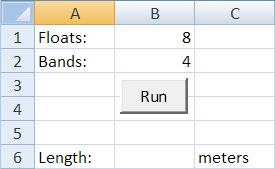The user types the number of floats and bands, and presses Run.

Add validation. Make sure that floats and bands are numbers, and zero or more. If the data is bad, show an error message in a `MsgBox`, and end the program. For example: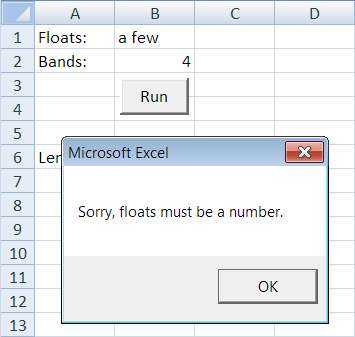If the input is OK, compute and output the result.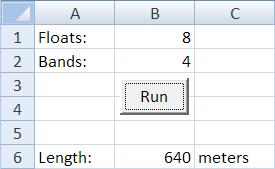Another example: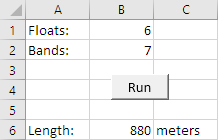Follow the usual coding standards for indenting and such.